Publicité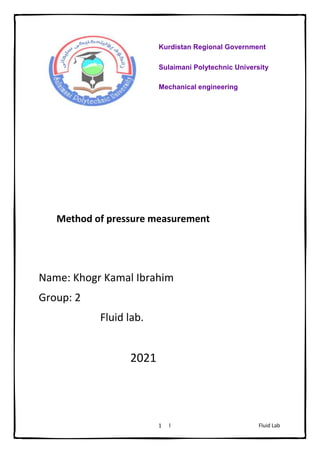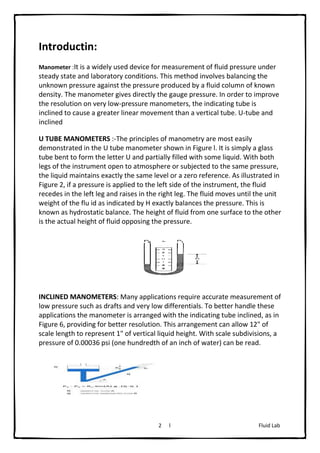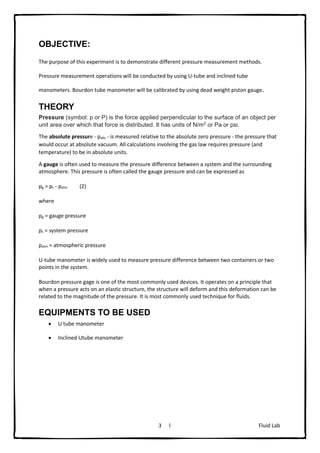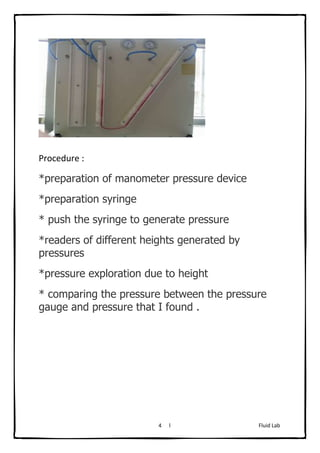Publicité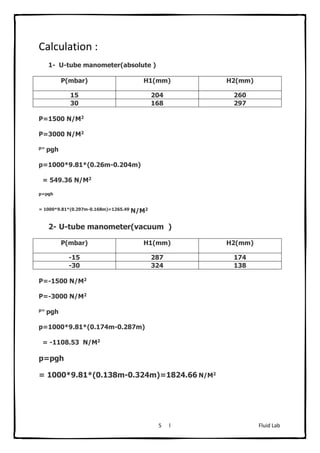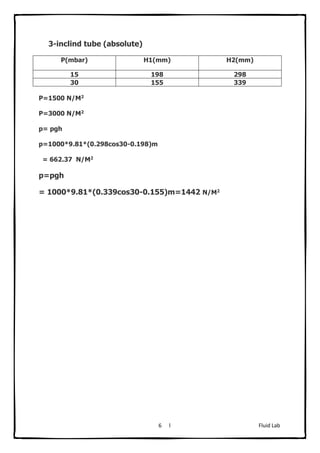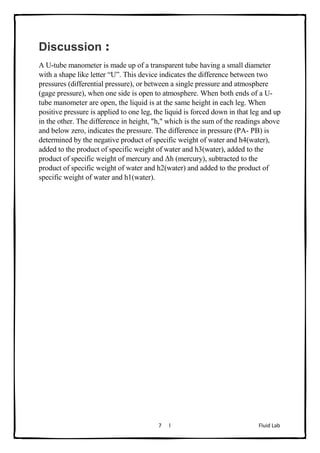Prochain SlideShare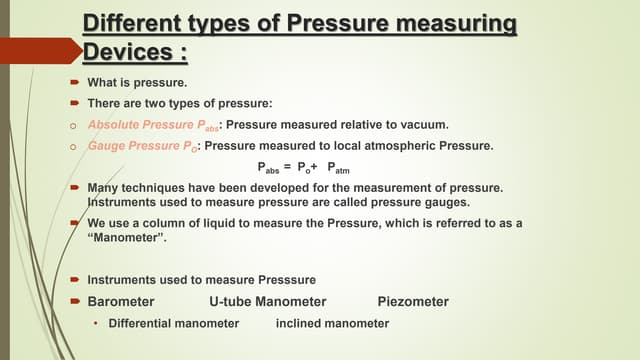Fluid mechanics
Chargement dans ... 3
1 sur 7
Publicité

### fluid lab presuure .docx

1. Fluid Lab l 1 Method of pressure measurement Name: Khogr Kamal Ibrahim Group: 2 Fluid lab. 2021 Kurdistan Regional Government Sulaimani Polytechnic University Mechanical engineering
2. Fluid Lab l 2 Introductin: Manometer :It is a widely used device for measurement of fluid pressure under steady state and laboratory conditions. This method involves balancing the unknown pressure against the pressure produced by a fluid column of known density. The manometer gives directly the gauge pressure. In order to improve the resolution on very low-pressure manometers, the indicating tube is inclined to cause a greater linear movement than a vertical tube. U-tube and inclined U TUBE MANOMETERS :-The principles of manometry are most easily demonstrated in the U tube manometer shown in Figure l. It is simply a glass tube bent to form the letter U and partially filled with some liquid. With both legs of the instrument open to atmosphere or subjected to the same pressure, the liquid maintains exactly the same level or a zero reference. As illustrated in Figure 2, if a pressure is applied to the left side of the instrument, the fluid recedes in the left leg and raises in the right leg. The fluid moves until the unit weight of the flu id as indicated by H exactly balances the pressure. This is known as hydrostatic balance. The height of fluid from one surface to the other is the actual height of fluid opposing the pressure. INCLINED MANOMETERS: Many applications require accurate measurement of low pressure such as drafts and very low differentials. To better handle these applications the manometer is arranged with the indicating tube inclined, as in Figure 6, providing for better resolution. This arrangement can allow 12" of scale length to represent 1" of vertical liquid height. With scale subdivisions, a pressure of 0.00036 psi (one hundredth of an inch of water) can be read.
3. Fluid Lab l 3 OBJECTIVE: The purpose of this experiment is to demonstrate different pressure measurement methods. Pressure measurement operations will be conducted by using U-tube and inclined tube manometers. Bourdon tube manometer will be calibrated by using dead weight piston gauge. THEORY Pressure (symbol: p or P) is the force applied perpendicular to the surface of an object per unit area over which that force is distributed. It has units of N/m2 or Pa or psi. The absolute pressure - pabs - is measured relative to the absolute zero pressure - the pressure that would occur at absolute vacuum. All calculations involving the gas law requires pressure (and temperature) to be in absolute units. A gauge is often used to measure the pressure difference between a system and the surrounding atmosphere. This pressure is often called the gauge pressure and can be expressed as pg = ps - patm (2) where pg = gauge pressure ps = system pressure patm = atmospheric pressure U-tube manometer is widely used to measure pressure difference between two containers or two points in the system. Bourdon pressure gage is one of the most commonly used devices. It operates on a principle that when a pressure acts on an elastic structure, the structure will deform and this deformation can be related to the magnitude of the pressure. It is most commonly used technique for fluids. EQUIPMENTS TO BE USED  U tube manometer  Inclined Utube manometer
4. Fluid Lab l 4 Procedure : *preparation of manometer pressure device *preparation syringe * push the syringe to generate pressure *readers of different heights generated by pressures *pressure exploration due to height * comparing the pressure between the pressure gauge and pressure that I found .
5. Fluid Lab l 5 Calculation : 1- U-tube manometer(absolute ) P(mbar) H1(mm) H2(mm) 15 204 260 30 168 297 P=1500 N/M2 P=3000 N/M2 p= pgh p=1000*9.81*(0.26m-0.204m) = 549.36 N/M2 p=pgh = 1000*9.81*(0.297m-0.168m)=1265.49 N/M2 2- U-tube manometer(vacuum ) P(mbar) H1(mm) H2(mm) -15 287 174 -30 324 138 P=-1500 N/M2 P=-3000 N/M2 p= pgh p=1000*9.81*(0.174m-0.287m) = -1108.53 N/M2 p=pgh = 1000*9.81*(0.138m-0.324m)=1824.66 N/M2
6. Fluid Lab l 6 3-inclind tube (absolute) P(mbar) H1(mm) H2(mm) 15 198 298 30 155 339 P=1500 N/M2 P=3000 N/M2 p= pgh p=1000*9.81*(0.298cos30-0.198)m = 662.37 N/M2 p=pgh = 1000*9.81*(0.339cos30-0.155)m=1442 N/M2
7. Fluid Lab l 7 Discussion : A U-tube manometer is made up of a transparent tube having a small diameter with a shape like letter “U”. This device indicates the difference between two pressures (differential pressure), or between a single pressure and atmosphere (gage pressure), when one side is open to atmosphere. When both ends of a U- tube manometer are open, the liquid is at the same height in each leg. When positive pressure is applied to one leg, the liquid is forced down in that leg and up in the other. The difference in height, "h," which is the sum of the readings above and below zero, indicates the pressure. The difference in pressure (PA- PB) is determined by the negative product of specific weight of water and h4(water), added to the product of specific weight of water and h3(water), added to the product of specific weight of mercury and Δh (mercury), subtracted to the product of specific weight of water and h2(water) and added to the product of specific weight of water and h1(water).
Publicité